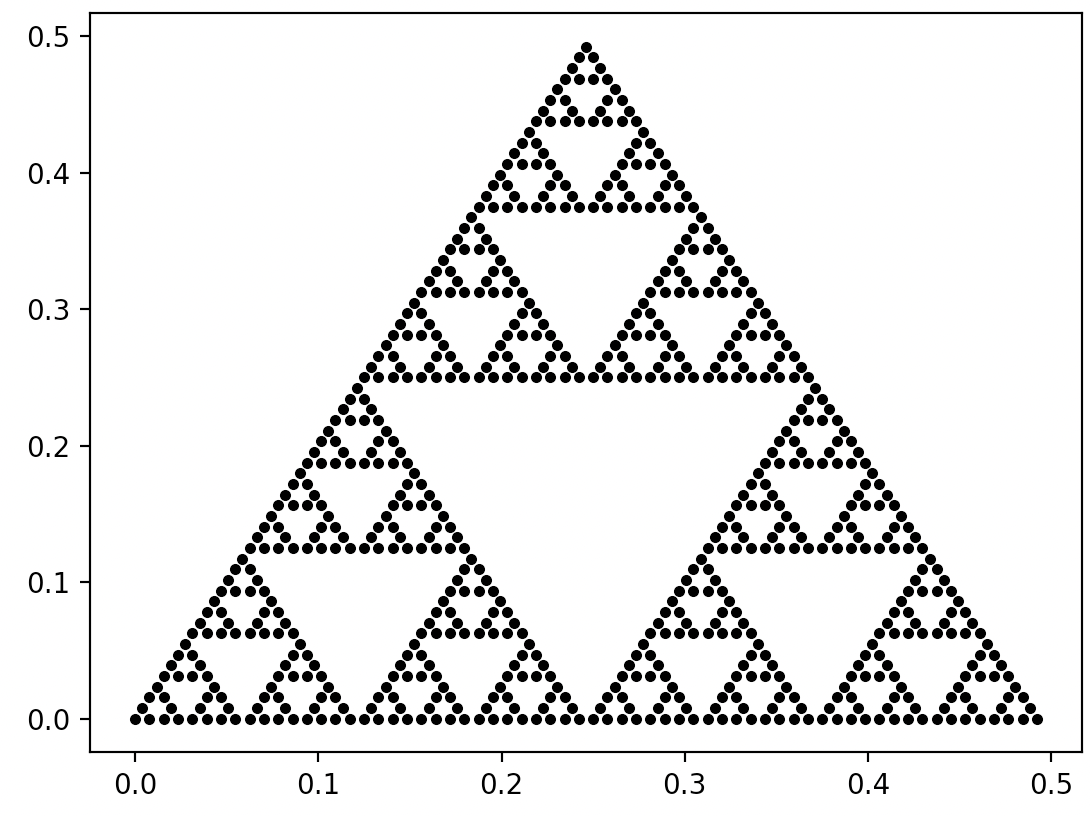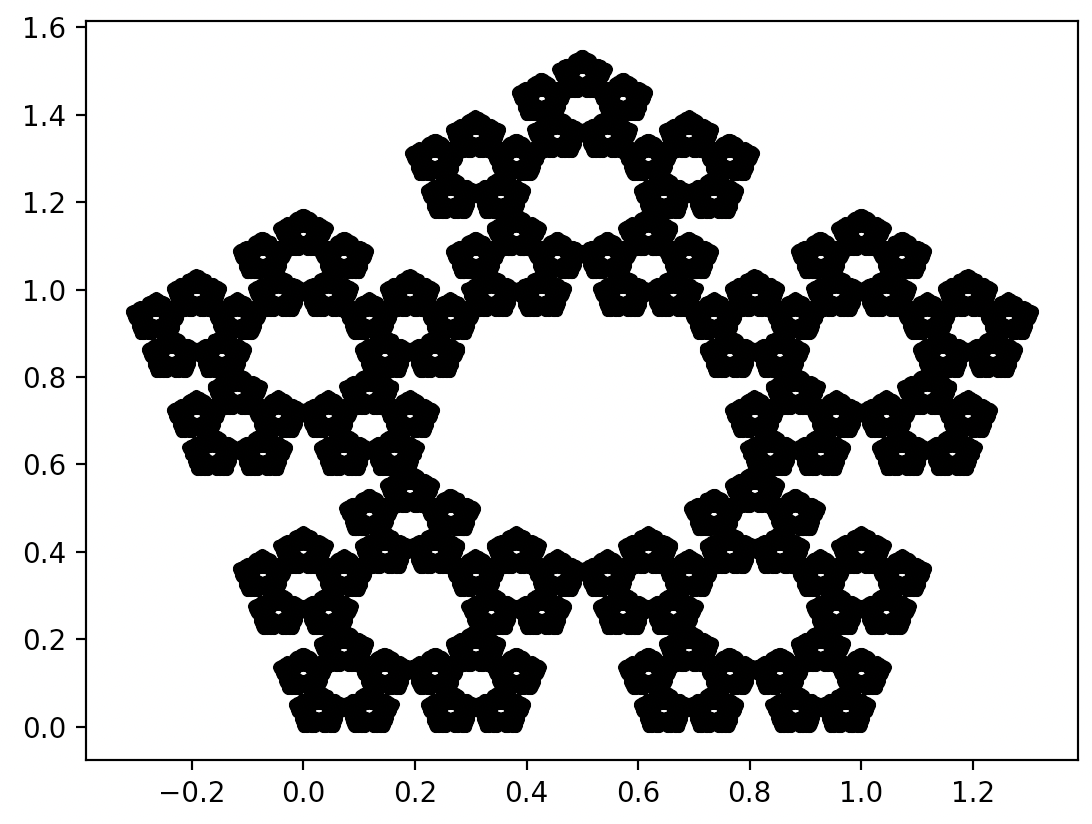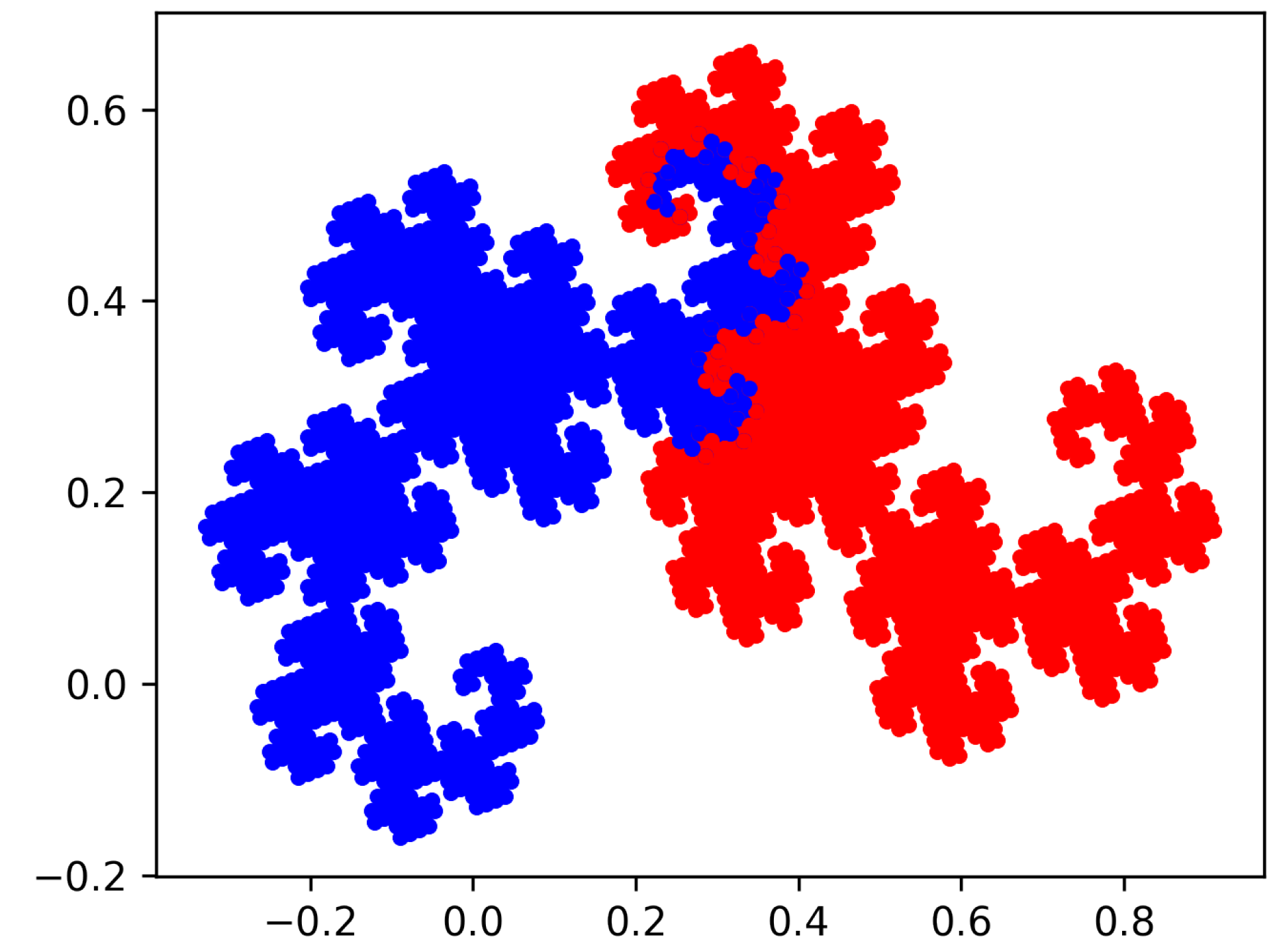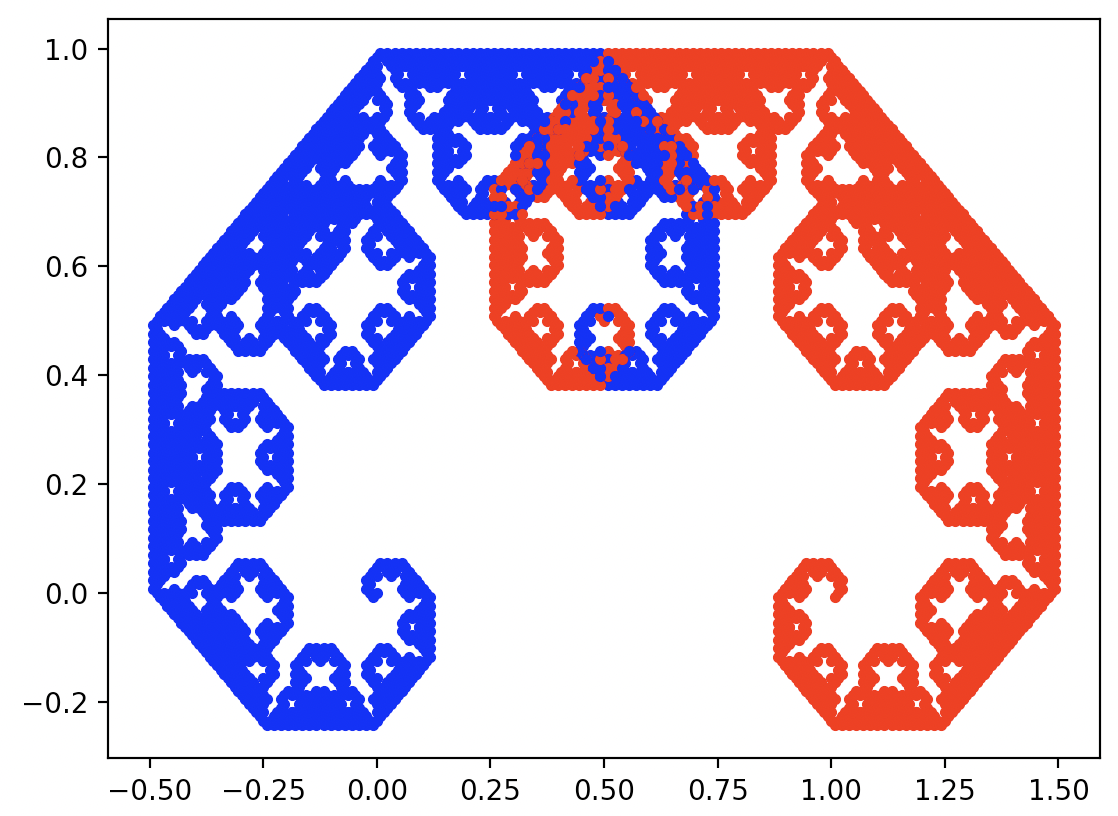## 2.4 Examples in Python

The examples presented in this chapter repeat the assembly of three atomic transformations – shift, rescale and rotate.

Below are the definitions of these three transformations. In the examples below, x is a two-element vector.

import numpy as np
import matplotlib.pyplot as plt

# Move point x by vector delta.
def shift(x, delta):

# Scale coordinates of point x by ratio.
def scale(x, ratio):
return np.multiply(x, ratio)

# Rotate by angle of alpha (in degrees).
def rotate(x, alpha):
adeg = math.pi * alpha / 180
rotation_matrix = [
return np.matmul(x, rotation_matrix)

### 2.4.1 Sierpinski triangle

As we wrote at the beginning of this chapter, fractals can be built from ordinary dots, we do not need more sophisticated polygons. Let’s introduce this on the basis of the Sierpiński triangle.

$y_1 = x * \left[\begin{smallmatrix} 0.5 & 0\\ 0 & 0.5 \end{smallmatrix}\right]$

$y_2 = x * \left[\begin{smallmatrix} 0.5 & 0\\ 0 & 0.5 \end{smallmatrix}\right] + \left[\begin{smallmatrix} 0.5 \\ 0 \end{smallmatrix}\right]$

$y_3 = x * \left[\begin{smallmatrix} 0.5 & 0\\ 0 & 0.5 \end{smallmatrix}\right] + \left[\begin{smallmatrix} 0.25 \\ \sqrt3/4 \end{smallmatrix}\right]$

The following program repeats the composition of these depth functions many times. In theory we would do this indefinitely, but it only takes a few steps to get a clear picture. The number of points grows exponentially, so after $$k$$ steps it’s equal to $$3^k$$.

import matplotlib.pyplot as plt
import numpy as np

# Move point x by vector delta.
def shift(x, delta):

# Scale coordinates of point x by ratio.
def scale(x, ratio):
return np.multiply(x, ratio)

def sierpinski(x, depth):
if depth > 1:
x1 = scale(shift(x,  [0, 0]), [0.5, 0.5])
sierpinski(x1, depth - 1)
x2 = scale(shift(x,  [0.5, 0]), [0.5, 0.5])
sierpinski(x2, depth - 1)
x3 = scale(shift(x,  [0.25, 0.5]), [0.5, 0.5])
sierpinski(x3, depth - 1)
else:
plt.plot(x, x, marker='o',
color="black", markersize=3)

# Initialize the drawing and draw the Sierpiński triangle.
plt.figure()
sierpinski([0,0], depth = 7)
plt.show()The result of executing the above instructions

### 2.4.2 Sierpinski pentagon

import math
import numpy as np
import matplotlib.pyplot as plt

# Move point x by vector delta.
def shift(x, delta):

# Scale coordinates of point x by ratio.
def scale(x, ratio):
return np.multiply(x, ratio)

def pentagon(x, depth, col="black"):
if depth > 1:
x1 = shift(scale(x, [0.382,0.382]), [0,0])
pentagon(x1, depth-1, col="red")
x2 = shift(scale(x, [0.382,0.382]), [0.618,0])
pentagon(x2, depth-1, col="blue")
x3 = shift(scale(x, [0.382,0.382]), [0.809,0.588])
pentagon(x3, depth-1, col="green")
x4 = shift(scale(x, [0.382,0.382]), [0.309,0.951])
pentagon(x4, depth-1, col="orange")
x5 = shift(scale(x, [0.382,0.382]), [-0.191,0.588])
pentagon(x5, depth-1, col="brown")
else:
plt.plot(x,x, marker='o', color=col, markersize=3)

plt.figure()
pentagon([0,0], depth=6, col)
plt.show()The result of executing the above instructions

### 2.4.3 Heighway’s Dragon

import math
import numpy as np
import matplotlib.pyplot as plt

import numpy as np
import matplotlib.pyplot as plt

# Move point x by vector delta.
def shift(x, delta):

# Scale coordinates of point x by ratio.
def scale(x, ratio):
return np.multiply(x, ratio)

# Rotate by angle of alpha (in degrees).
def rotate(x, alpha):
adeg = math.pi * alpha / 180
rotation_matrix = [
return np.matmul(x, rotation_matrix)

def heighway(x, depth, col="black"):
if depth > 1:
x1 = rotate(x, -45)
x1 = scale(x1, [np.sqrt(0.5), np.sqrt(0.5)])
heighway(x1, depth-1, col="blue")
x2 = rotate(x, -135)
x2 = scale(x2, [np.sqrt(0.5), np.sqrt(0.5)])
x2 = shift(x2, [0.75, 0.25])
heighway(x2, depth-1, col="red")
else:
plt.plot(x, x, marker='o', color=col, markersize=3)

plt.figure()
heighway([0,0], depth=14)
plt.show()The result of executing the above instructions

### 2.4.4 Symmetric binary tree / Pythagoras’ tree

import numpy as np
import matplotlib.pyplot as plt

# Move point x by vector delta.
def shift(x, delta):

# Scale coordinates of point x by ratio.
def scale(x, ratio):
return np.multiply(x, ratio)

# Rotate by angle of alpha (in degrees).
def rotate(x, alpha):
adeg = math.pi * alpha / 180
rotation_matrix = [
return np.matmul(x, rotation_matrix)

def sbt(x, depth, col="black"):
if depth > 1:
x1 = rotate(x, -45)
x1 = shift(scale(x1, [0.7, 0.7]), [0, 1])
sbt(x1, depth-1, col="blue")
x2 = rotate(x, 45)
x2 = shift(scale(x2, [0.7, 0.7]), [0, 1])
sbt(x2, depth-1, col="red")
else:
plt.plot(x, x, marker='o', color=col, markersize=3)

plt.figure()
sbt([0,0], depth = 14)
plt.show()The result of executing the above instructions